Courses

# Long Answer Questions - Consumer's Equilibrium and Demand (Theory of Consumer Behaviour) Commerce Notes | EduRev

## Commerce : Long Answer Questions - Consumer's Equilibrium and Demand (Theory of Consumer Behaviour) Commerce Notes | EduRev

The document Long Answer Questions - Consumer's Equilibrium and Demand (Theory of Consumer Behaviour) Commerce Notes | EduRev is a part of the Commerce Course Economics Class 12.
All you need of Commerce at this link: Commerce

Q.1. How is equilibrium achieved with the help of indifference curve analysis?
Ans :- a) Definition: In the indifference curve approach, consumer’s equilibrium is achieved at the point at which the budget line is tangent to a particular indifference curve. This is the point of maximum satisfaction.
b) Diagram: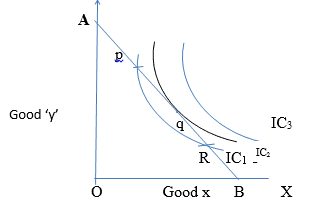c) Explanation of the diagram:
i) ‘AB’ is the budget line.
ii) It is sure that consumer’s equilibrium will lie on some point on ‘AB’
iii) Indifference map (set of IC1, IC2 , IC3) shows consumers scale of preferences between different combinations of good ‘x’ and good ‘y’
iv) Consumers’ equilibrium will achieve where budget line (AB) is tangent to the IC2.
d) Essential conditions for consumers equilibrium:
i) Budget line must be tangent to indifference curve i.e., MRS xy = Px / Py
ii) Indifference curve must be convex to the origin or MRS xy should decrease.
e) Consumers cannot achieve the following:
i) P and R points on budget line give satisfaction but they lie on lower indifference curve IC1. Choosing point ‘q’ puts him on a higher IC which gives more satisfaction.
ii) He cannot move on IC3, as it is beyond his money income.

Q.2. Explain the factors affecting the market demand of a commodity.
Ans :- i) Meaning: Market demand is the aggregates of the quantities demanded by all the consumers in the market at different prices.
ii) Factors affecting market demand :

1. Price of the commodity: When the price goes up demand for its falls and vice-versa.
2. Income of the consumers: When the income of the consumers goes up the demand for a commodity also goes up.
3. Price of related goods :
a. Complementary goods :The demand for a commodity rises with a fall in the price of its complementary good (Car and petrol)
b. Substitute goods: Demand for a commodity falls with a fall in the price of other substitute good (Tea & Coffee).
4. Tastes and preferences: Any favourable change in consumers’ tastes will lead to increase in market demand and any unfavourable change in consumers tastes will lead to decrease in market demand.
5. Consumer’s group: More the consumers more will be market demand and vice-versa.

Q.3. Explain the various degrees of price elasticity of demand with the help of diagrams.

Ans:- There are five degrees of price elasticity of demand. They are,

1. Perfectly elastic demand (Ed=∞):- a slight or no change in the price leads to infinite changes in the quantity demanded.
2. Perfectly Inelastic demand (Ed=0) :- Demand of a commodity does not change at all irrespective of any change in its price.
3. Unitary elastic demand (Ed=1):- When the percentage change in demand (%) of a commodity is equal to the percentage change in price.
4. Greater than unitary elastic demand (Ed>1):- When percentage change in demand of a commodity is more than the percentage change in its price.
5. Less than unitary elastic demand (Ed<1) :- When percentage change in demand of a commodity is less than the percentage change in its price.

Diagrams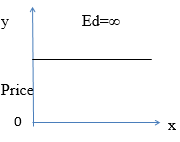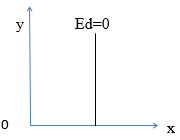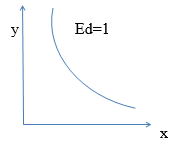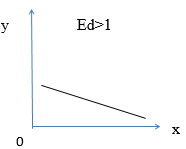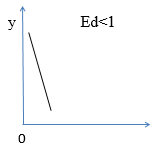Numerical for practice

Derive the total utility schedule from the marginal utility.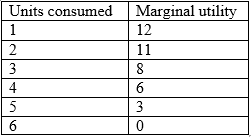Q.4. A consumer buys 50 units of a good at Rs. 4/- per unit. When its price falls by 25 percent its demand rises to 100 units. Find out the price elasticity of demand.
Ans:- Ed=4

Q.5. Price elasticity of demand for wheat is equal to unity and a household demands 40 Kg of wheat when the price is Rs.1 per kg. At what price will the household demand 36 kg of wheat?
Ans:- The price of wheat rises to Rs.1.10 per kg.

Q.6. The quantity demanded of a commodity at a price of Rs.10 per unit is 40 units. Its price elasticity of demand is -2. Its price falls by Rs.2/- per unit. Calculate its quantity demanded at the new price.
Ans :- 56 units.

## Economics Class 12

225 videos|237 docs|54 tests

,

,

,

,

,

,

,

,

,

,

,

,

,

,

,

,

,

,

,

,

,

;